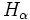# Hypernormalized subgroup

This article defines a subgroup property: a property that can be evaluated to true/false given a group and a subgroup thereof, invariant under subgroup equivalence. View a complete list of subgroup properties[SHOW MORE]
This is a variation of normality|Find other variations of normality | Read a survey article on varying normality

## Definition

### Symbol-free definition

A subgroup of a group is said to be hypernormalized if its hypernormalizer is the whole group. Here, the hypernormalizer is the limit of the transfinite ascending chain that begins with the subgroup and where each successor is the normalizer of its predecessor in the whole group.

If the hypernormalizer sequence reachse the whole group in finitely many steps, we call the subgroup finitarily hypernormalized.

### Definition with symbols

A subgroup$H$ of a group$G$ is said to be hypernormalized if the sequence$H_{\alpha}$ ends at$G$, where <math.H_\alpha[/itex] is defined as follows:

•$H_0 = H$
•$H_{\alpha + 1} = N_G(H_{\alpha})$
•$H_\alpha$ is the ascending chain union of$H_\beta$ for$\beta < \alpha$ when$\alpha$ is a limit ordinal

If$H_r = G$ for a finite integer$r$, we say that$H$ is finiarily hypernormalized in$G$.

## Relation with other properties

### Related group properties

• HN-group is a group where every subgroup is hypernormalized.

## Metaproperties

### Transitivity

NO: This subgroup property is not transitive: a subgroup with this property in a subgroup with this property, need not have the property in the whole group
ABOUT THIS PROPERTY: View variations of this property that are transitive|View variations of this property that are not transitive
ABOUT TRANSITIVITY: View a complete list of subgroup properties that are not transitive|View facts related to transitivity of subgroup properties | View a survey article on disproving transitivity

A hypernormalized subgroup of a hypernormalized subgroup need not be hypernormalized. This can be seen from the fact that there are subnormal subgroups which are not hypernormalized.